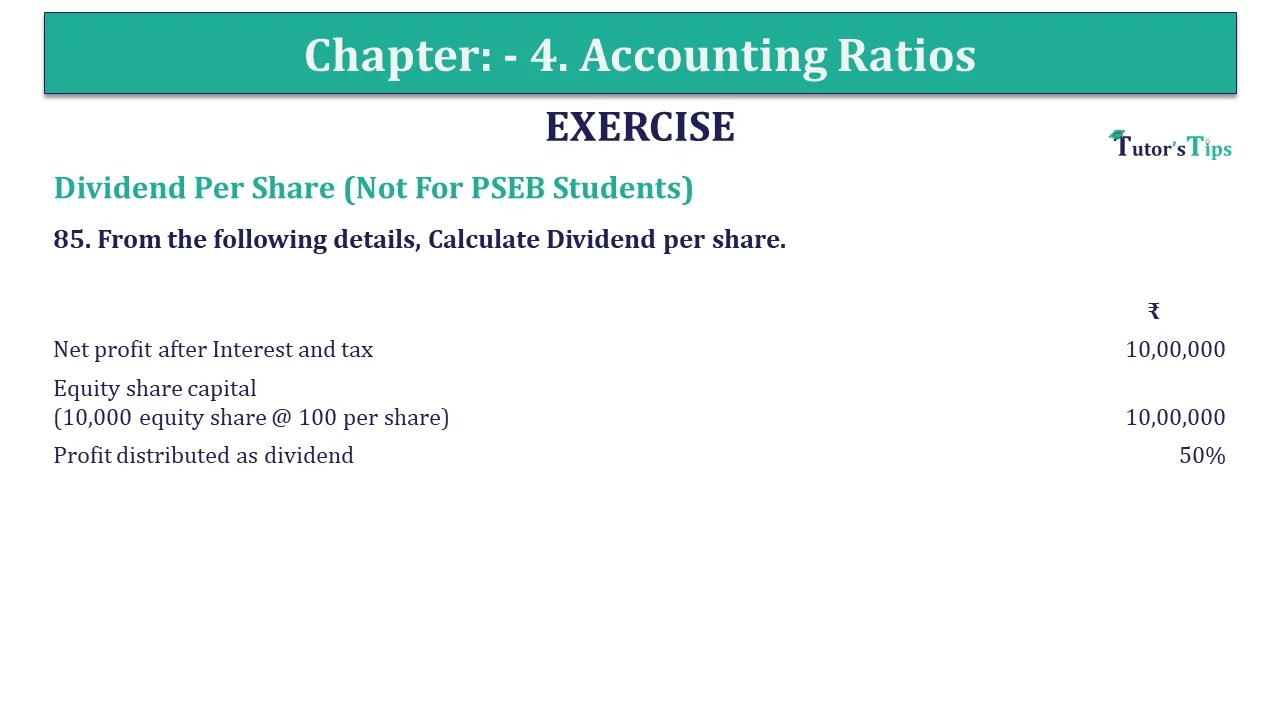# Question 85 Chapter 4 of +2-B – USHA Publication 12 ClassQ-85- CH-4 Book 2 - Usha Pub. +2 Book 2020 - Solution

Question 85 Chapter 4 of +2-B

Earning Per Share (Not For PSEB Students)

85. From the following details, Calculate the Dividend per share.

 ₹ Net profit after Interest and tax 10,00,000 Equity share capital 10,00,000 (10,000 equity share @ 100 per share) Profit distributed as dividend 50%

## The solution of Question 85 Chapter 4 of +2-B: –

 Return On Capital Employed Ratio = Net profit after Interest and Tax Preference Dividend Number of Equity Shares = = ₹ 5,00,000 ₹ 10,000 = ₹ 50

 Profit Distributed as equity Dividend = 50% of Equity Share Capital = 50 X ₹ 10,00,000 100 = ₹ 5,00,000

Also, Check out the solved question of previous Chapters: –